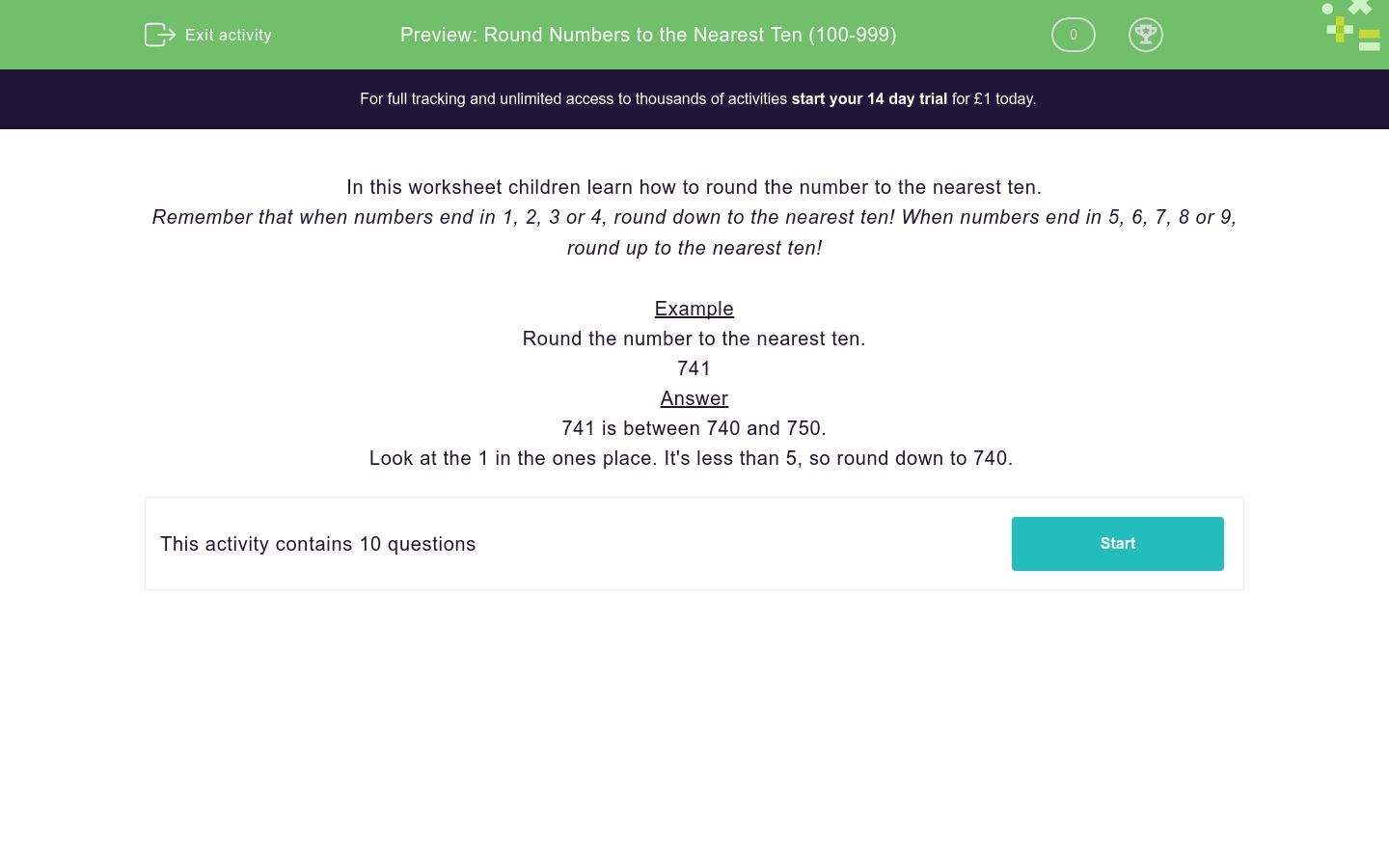# Round Numbers to the Nearest Ten (100-999)

In this worksheet, students round numbers to the nearest ten.Key stage:  KS 2

Curriculum topic:   Number: Number and Place Value

Curriculum subtopic:   Round Numbers Up/Down (to 10, 100 or 1000)

Difficulty level:### QUESTION 1 of 10

In this worksheet children learn how to round the number to the nearest ten.

Remember that when numbers end in 1, 2, 3 or 4, round down to the nearest ten! When numbers end in 5, 6, 7, 8 or 9, round up to the nearest ten!

Example

Round the number to the nearest ten.

741

741 is between 740 and 750.

Look at the 1 in the ones place. It's less than 5, so round down to 740.

Round the number to the nearest ten.

155

Round the number to the nearest ten.

876

Round the number to the nearest ten.

872

Round the number to the nearest ten.

515

Round the number to the nearest ten.

983

Round the number to the nearest ten.

268

Round the number to the nearest ten.

962

Round the number to the nearest ten.

225

Round the number to the nearest ten.

351

Round the number to the nearest ten.

958

• Question 1

Round the number to the nearest ten.

155

160
EDDIE SAYS
155 is in between 150 and 160. There is a 5 in the ones place, so round up to 160.
• Question 2

Round the number to the nearest ten.

876

880
EDDIE SAYS
876 is in between 870 and 880. There is a 6 in the ones place, so round up to 880.
• Question 3

Round the number to the nearest ten.

872

870
EDDIE SAYS
872 is in between 870 and 880. There is a 2 in the ones place, so round down to 870.
• Question 4

Round the number to the nearest ten.

515

520
EDDIE SAYS
515 is in between 510 and 520. There is a 5 in the ones place, so round up to 520.
• Question 5

Round the number to the nearest ten.

983

980
EDDIE SAYS
983 is in between 980 and 990. There is a 3 in the ones place, so round down to 980.
• Question 6

Round the number to the nearest ten.

268

270
EDDIE SAYS
268 is in between 260 and 270. There is an 8 in the ones place, so round up to 270.
• Question 7

Round the number to the nearest ten.

962

960
EDDIE SAYS
962 is in between 960 and 970. There is a 2 in the ones place, so round down to 960.
• Question 8

Round the number to the nearest ten.

225

230
EDDIE SAYS
225 is in between 220 and 230. There is a 5 in the ones place, so round up to 230.
• Question 9

Round the number to the nearest ten.

351

350
EDDIE SAYS
351 is in between 350 and 360. There is a 1 in the ones place, so round down to 350.
• Question 10

Round the number to the nearest ten.

958

960
EDDIE SAYS
958 is in between 950 and 960. There is an 8 in the ones place, so round up to 960.
---- OR ----

Sign up for a £1 trial so you can track and measure your child's progress on this activity.

### What is EdPlace?

We're your National Curriculum aligned online education content provider helping each child succeed in English, maths and science from year 1 to GCSE. With an EdPlace account you’ll be able to track and measure progress, helping each child achieve their best. We build confidence and attainment by personalising each child’s learning at a level that suits them.

Get started